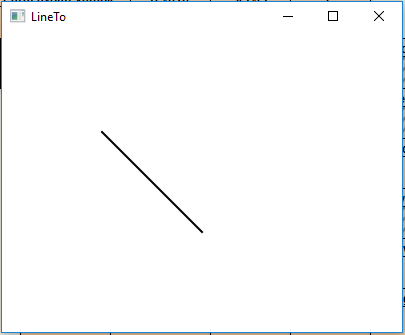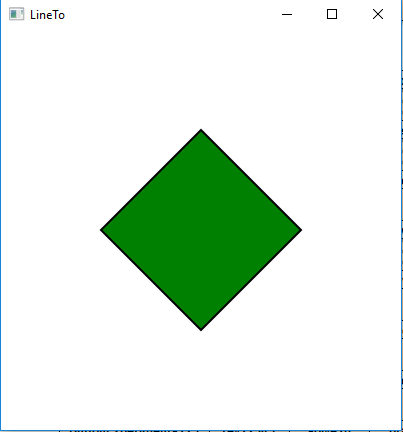# JavaFX | LineTo class

LineTo class is a part of JavaFX. LineTo class draws a line from the current position to specified x and y coordinate. LineTo class inherits PathElement class.

Constructor of the class:

1. LineTo(): Creates a new LineTo object.
2. LineTo(double x, double y): Creates a new LineTo object with specified x, y coordinates.

Commonly Used Methods:

Method Explanation
getX() Returns the value of X coordinate.
getY() Returns the value of Y coordinate.
setX(double v) Sets the value of X coordinate.
setY(double v) Sets the value of Y coordinate.
xProperty() Defines the X coordinate.
yProperty() Defines the Y coordinate.

Below programs illustrate the use of LineTo Class:

1. Java program to create a path and add LineTo object to it and display it:
• In this program, we will create a Path object named path.
• Create an HLineTo object with specified X and Y coordinate.
• Then create a MoveTo object named moveto.
• Now add the MoveTo and Lineto object to the path.
• Add this path to Group object and add the group object to the scene and add the scene to the stage and call the show() function to display the final results.

 `// Java program to create a path and ` `// add LineTo object to it and display it ` `import` `javafx.application.Application; ` `import` `javafx.scene.Scene; ` `import` `javafx.scene.control.*; ` `import` `javafx.scene.layout.*; ` `import` `javafx.stage.Stage; ` `import` `javafx.scene.layout.*; ` `import` `javafx.scene.paint.*; ` `import` `javafx.scene.text.*; ` `import` `javafx.geometry.*; ` `import` `javafx.scene.layout.*; ` `import` `javafx.scene.shape.*; ` `import` `javafx.scene.paint.*; ` `import` `javafx.scene.*; ` ` `  `public` `class` `LineTo_1 ``extends` `Application { ` ` `  `    ``// launch the application ` `    ``public` `void` `start(Stage stage) ` `    ``{ ` ` `  `        ``try` `{ ` ` `  `            ``// set title for the stage ` `            ``stage.setTitle(``"LineTo"``); ` ` `  `            ``// create LineTo ` `            ``LineTo Lineto = ``new` `LineTo(``200``, ``200``); ` ` `  `            ``// create moveto ` `            ``MoveTo moveto = ``new` `MoveTo(``100``, ``100``); ` ` `  `            ``// create a Path ` `            ``Path path = ``new` `Path(moveto, Lineto); ` ` `  `            ``// set fill for path ` `            ``path.setFill(Color.BLACK); ` ` `  `            ``// set stroke width ` `            ``path.setStrokeWidth(``2``); ` ` `  `            ``// create a Group ` `            ``Group group = ``new` `Group(path); ` ` `  `            ``// create a scene ` `            ``Scene scene = ``new` `Scene(group, ``400``, ``300``); ` ` `  `            ``// set the scene ` `            ``stage.setScene(scene); ` ` `  `            ``stage.show(); ` `        ``} ` ` `  `        ``catch` `(Exception e) { ` ` `  `            ``System.out.println(e.getMessage()); ` `        ``} ` `    ``} ` ` `  `    ``// Main Method ` `    ``public` `static` `void` `main(String args[]) ` `    ``{ ` ` `  `        ``// launch the application ` `        ``launch(args); ` `    ``} ` `} `

Output:2. Java program to create a path and add multiple LineTo objects to it and display it:
• In this program, we will create a Path object named path.
• Create four LineTo object with specified X and Y coordinates names Lineto, Lineto1, Lineto2, Lineto3.
• Then create a MoveTo object named moveto.
• Now add the MoveTo and Lineto objects to the path.
• Add this path to Group object and add the group object to the scene and add the scene to the stage and call the show() function to display the final results.

 `// Java program to create a path and ` `// add  multiple LineTo objects to it ` `// and display it ` `import` `javafx.application.Application; ` `import` `javafx.scene.Scene; ` `import` `javafx.scene.control.*; ` `import` `javafx.scene.layout.*; ` `import` `javafx.stage.Stage; ` `import` `javafx.scene.layout.*; ` `import` `javafx.scene.paint.*; ` `import` `javafx.scene.text.*; ` `import` `javafx.geometry.*; ` `import` `javafx.scene.layout.*; ` `import` `javafx.scene.shape.*; ` `import` `javafx.scene.paint.*; ` `import` `javafx.scene.*; ` ` `  `public` `class` `LineTo_2 ``extends` `Application { ` ` `  `    ``// launch the application ` `    ``public` `void` `start(Stage stage) ` `    ``{ ` ` `  `        ``try` `{ ` ` `  `            ``// set title for the stage ` `            ``stage.setTitle(``"LineTo"``); ` ` `  `            ``// create LineTo ` `            ``LineTo Lineto = ``new` `LineTo(``300``, ``200``); ` `            ``LineTo Lineto1 = ``new` `LineTo(``200``, ``300``); ` `            ``LineTo Lineto2 = ``new` `LineTo(``100``, ``200``); ` `            ``LineTo Lineto3 = ``new` `LineTo(``200``, ``100``); ` ` `  `            ``// create moveto ` `            ``MoveTo moveto = ``new` `MoveTo(``200``, ``100``); ` ` `  `            ``// create a Path ` `            ``Path path = ``new` `Path(moveto, Lineto,  ` `                     ``Lineto1, Lineto2, Lineto3); ` ` `  `            ``// set fill for path ` `            ``path.setFill(Color.GREEN); ` ` `  `            ``// set stroke width ` `            ``path.setStrokeWidth(``2``); ` ` `  `            ``// create a Group ` `            ``Group group = ``new` `Group(path); ` ` `  `            ``// create a scene ` `            ``Scene scene = ``new` `Scene(group, ``400``, ``400``); ` ` `  `            ``// set the scene ` `            ``stage.setScene(scene); ` ` `  `            ``stage.show(); ` `        ``} ` ` `  `        ``catch` `(Exception e) { ` ` `  `            ``System.out.println(e.getMessage()); ` `        ``} ` `    ``} ` ` `  `    ``// Main Method ` `    ``public` `static` `void` `main(String args[]) ` `    ``{ ` ` `  `        ``// launch the application ` `        ``launch(args); ` `    ``} ` `} `

Output:Note: The above programs might not run in an online IDE please use an offline compiler.

Attention reader! Don’t stop learning now. Get hold of all the important DSA concepts with the DSA Self Paced Course at a student-friendly price and become industry ready.

My Personal Notes arrow_drop_upCheck out this Author's contributed articles.

If you like GeeksforGeeks and would like to contribute, you can also write an article using contribute.geeksforgeeks.org or mail your article to contribute@geeksforgeeks.org. See your article appearing on the GeeksforGeeks main page and help other Geeks.

Please Improve this article if you find anything incorrect by clicking on the "Improve Article" button below.

Article Tags :
Practice Tags :

Be the First to upvote.

Please write to us at contribute@geeksforgeeks.org to report any issue with the above content.